# High School Math : Polynomials

## Example Questions

1 2 4 Next →

### Example Question #31 : Intermediate Single Variable Algebra

Factor the following polynomial: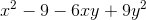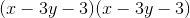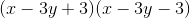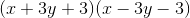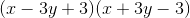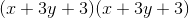Explanation:

Begin by rearranging the terms to group together the quadratic: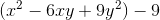Now, convert the quadratic into a square: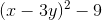Finally, distribute the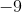:### Example Question #32 : Intermediate Single Variable Algebra

Factor the following polynomial: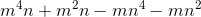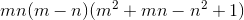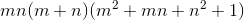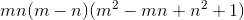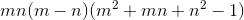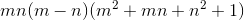Explanation:

Begin by extracting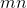from the polynomial: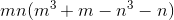Now, rearrange to combine like terms: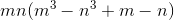Extract the like terms and factor the cubic: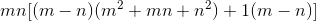Simplify by combining like terms:### Example Question #33 : Intermediate Single Variable Algebra

Factor the following polynomial: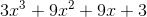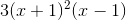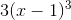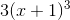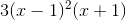Explanation:

Begin by extractingfrom the polynomial: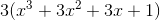Now, rearrange to combine like terms: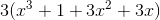Extract the like terms and factor the cubic: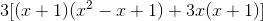Simplify by combining like terms: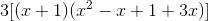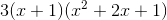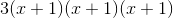### Example Question #1 : Write A Polynomial Function From Its Zeros

Consider the equation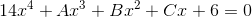.

According to the Rational Zeroes Theorem, if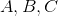are all integers, then, regardless of their values, which of the following cannot be a solution to the equation?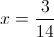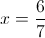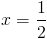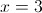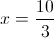Explanation:

By the Rational Zeroes Theorem, any rational solution must be a factor of the constant, 6, divided by the factor of the leading coefficient, 14.

Four of the answer choices have this characteristic: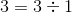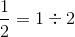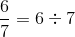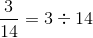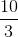is in lowest terms, and 3 is not a factor of 14. It is therefore the correct answer.

1 2 4 Next →